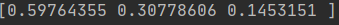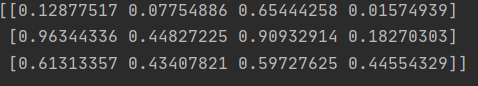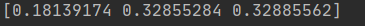• python创建数组对象
2021-04-26 19:01:19

Numpy提供了很多方式(函数)来创建数组对象，常用的方式如下：

•array

•arange

•ones / ones_like

•zeros / zeros_like

•empty / empty_like

•full / full_like

•eye / identity

•linspace

•logspace

•frombuffer

•fromiter

•fromfunction

说明：

•注意arange函数，不是arrange。

•arange与linspace的区别。

1、array实例

def npsum():

a=np.array([1,2,3,4,5])

b=np.array([2,3,4,1,2])

c=a

2+b

2

return c

print(npsum())

2、python中的range()函数生成list

语法：range(start, end, step) 指定起始范围和步长

步长不能为浮点

3、arange功能更强大：步长可以是浮点数，也可以是负数

4、创建值全为1的数组。

n = np.ones((3, 4)) # 生成一个 3行4列的 元素全部都是 1 的一个二维数组

display(n)

创建值全为0的数组。

n = np.zeros((3, 4))

display(n)

创建值全为-8.8的数组。

n = np.full((3, 4), -8.8) # 第一个参数；维度， 第二个参数；填充的元素的值

display(n)

array([[-8.8, -8.8, -8.8, -8.8],

[-8.8, -8.8, -8.8, -8.8],

[-8.8, -8.8, -8.8, -8.8]])

更多相关内容
• 间接定义matrix=[0foriinrange(4)]print(matrix)相关推荐：《Python基础教程》二、Numpy方法：Numpy内置了从头开始创建数组的函数：zeros(shape)将创建一个用指定形状用0填充的数组。默认的dtype是float64。下面是几...一、直接定义法：

1.直接定义matrix=[0,1,2,3]

2.间接定义matrix=[0 for i in range(4)]

print(matrix)

相关推荐：《Python基础教程》

二、Numpy方法：

Numpy内置了从头开始创建数组的函数：zeros(shape)将创建一个用指定形状用0填充的数组。默认的dtype是float64。

下面是几种常用的创建方法：#coding=utf-8

import numpy as np

a = np.array([1,2,3,4,5])

print a

b = np.zeros((2,3))

print b

c = np.arange(10)

print c

d = np.arange(2,10,dtype=np.float)

print d

e = np.linspace(1.0,4.0,6)

print e

f = np.indices((3,3))

print f

三、其他转换法：

数组还有比较常用的一种方法，就是从其他Python结构(例如，列表，元组)转换。

下面给出一些例子。

列表转数组：a = []

a.append((1,2,4))

a.append((2,3,4))

a = np.array(a)

a.flatten()

元组转成数组：import numpy as np

mylist = [1,2,3]

print tuple(mylist)

iarray = np.array(tuple(mylist))

print iarray

展开全文• ## python创建数组

万次阅读 2021-04-25 22:29:12
NumPy模块提供了多种创建数组的方法，创建的数组类型也有多种多样，下面列举一下创建数组的方法： 1.使用array()函数创建数组 函数可基于序列型的对象（如列表、元组、集合等，还可以是一个已经创建好的数组） #...

NumPy模块最主要的特点就是引入了数组的概念。数组是一些相公类型的数据集合，这些数据按照一定的顺序排列，并且每个数据占用大小相同的存储空间。要使用数组组织数据，首先就要创建数组。NumPy模块提供了多种创建数组的方法，创建的数组类型也有多种多样，下面列举一下创建数组的方法：

1.使用array()函数创建数组
函数可基于序列型的对象（如列表、元组、集合等，还可以是一个已经创建好的数组）

#使用array函数创建数组
import numpy as np  #导入numpy模块，用as可以起别名
a=np.array([1,2,3,4])
b=np.array(['产品编号','销售数量','销售单价','销售金额'])
print(a)


1.1创建二维数组

import numpy as np
a=np.array([[1,2,3],[4,5,6],[7,8,9]])
print(a)


2.创建等差数组
用arange()函数创建数组会更加方便

import numpy as np
d=np.arange(1,20,3)#起始值是1，结束值是20（结果不含该值），步长为1
print(d)


3.创建随机数组
引用numpy模块的子模块random中的函数，主要有rand()函数、randn()函数、randint()函数。
3.1rand（）函数创建的数组中每个元素都是[0,1)区间内的随机数

import numpy as np
e=np.random.rand(3)
print(e)如果给rand()函数传入一对参数值，就会生成一个相应行、列数的二维数组，区间也是[0,1)的随机数

import numpy as np
e=np.random.rand(3,4)
print(e)3.2randn()函数
用randn()函数创建的数组中的元素是符合标准正态分布（均值为0，标准差为1）的随机数

import numpy as np
e=np.random.rand(3,4)
print(e)3.3randint()函数
用randint()函数创建的数组中元素是制定范围内的随机整数

import numpy as np
e = np.random.randint(1,5,10) #10个元素区间在[1,5)区间内的随机整数，随机数不包括5
print(e)


今天就先分享这么多了，睡觉了，各位晚安

展开全文• 间接定义matrix=[0 for i in range(4)]print(matrix)二、Numpy方法：Numpy内置了从头开始创建数组的函数：zeros(shape)将创建一个用指定形状用0填充的数组。默认的dtype是float64。下面是几种常用的创建方法：#...

原标题：python怎么创建对象数组?一、直接定义法：

1.直接定义

matrix=[0,1,2,3]

2.间接定义

matrix=[0 for i in range(4)]

print(matrix)

二、Numpy方法：

Numpy内置了从头开始创建数组的函数：zeros(shape)将创建一个用指定形状用0填充的数组。默认的dtype是float64。

下面是几种常用的创建方法：

#coding=utf-8

import numpy as np

a = np.array([1,2,3,4,5])

print a

b = np.zeros((2,3))

print b

c = np.arange(10)

print c

d = np.arange(2,10,dtype=np.float)

print d

e = np.linspace(1.0,4.0,6)

print e

f = np.indices((3,3))

print f

三、其他转换法：

数组还有比较常用的一种方法，就是从其他Python结构(例如，列表，元组)转换。

下面给出一些例子。

列表转数组：

a = []

a.append((1,2,4))

a.append((2,3,4))

a = np.array(a)

a.flatten()

元组转成数组：

import numpy as np

mylist = [1,2,3]

print tuple(mylist)

iarray = np.array(tuple(mylist))

print iarray

原文至：https://www.py.cn/jishu/jichu/13321.html返回搜狐，查看更多

责任编辑：

展开全文• ## Python创建数组

千次阅读 2021-10-22 10:22:07
创建数组有5种常规机制： 从其他Python结构（例如，列表，元组）转换 numpy原生数组的创建（例如，arange、ones、zeros等） 从磁盘读取数组，无论是标准格式还是自定义格式 通过使用字符串或缓冲区从原始字节创建...python
• 二：python类与对象 2.1创建类 类中的函数称为方法；你前面学到的有关函数的一切都适用于方法，就目前而言，唯一重要的差别是调用方法的方式。❸处的方法__init__() 是一个特殊的方法，每当你根 据Dog 类创建新...python
• Numpy内置了从头开始创建数组的函数：zeros(shape)将创建一个用指定形状用0填充的数组。默认的dtype是float64。 下面是几种常用的创建方法： #coding=utf-8 import numpy as np a = np.array([1,2,3,4,5]) ...python 机器学习
• 计算机为数组分配一段连续的内存，从而...一个项的偏移地址就等于它的索引乘以数组的一个项所需要的内存单元数目的一个常量表示(在python中，这个值总是1)import array#array模块是python中实现的一种高效的数组存...
• 1.数组对象基础ndarray是NumPy的核心功能，其含义是：n-...2.初识数组对象在Jupyter中查看NumPy的版本(按shift + enter即可运行代码)import numpy as npnp.__version__输出结果如下：2.1 创建第一个数组In...
• 本文实例讲述了Python使用numpy模块创建数组操作。分享给大家供大家参考，具体如下： 创建数组 创建ndarray 创建数组最简单的方法就是使用array函数。它接收一切序列型的对象（包括其他数组），然后产生一个新的含有...
• ## 从Python列表创建数组

千次阅读 热门讨论 2021-11-10 20:19:46python
• 广告关闭腾讯云11.11云上盛惠 ，精选热门产品助力上云... numpy模块提供了一种新的python数据结构——数组(array)，以及特定于该结构的函数工具箱。 该模块还支持随机数、数据聚合、线性代数和傅里叶变换等非常实用...python建立数组
• 我的做法是先将数据保存在dict内,再将dict插入到数组,最后通过json.dumps()将数据压缩为Json格式,具体数据流图如下:详细代码:detail = []for station in stations.find_all("td"):if station.text.strip():detail....
• python填充数组的外围填充 matrix_pad = np.pad(matrix_arr, pad_width=((1, 1), # 向上填充1个维度，向下填充1个维度 (1, 1)), # 向左填充1个维度，向右填充1个维度 mode="constant", # 填充模式 constant_...python
• ## python读入数组

千次阅读 2020-11-23 14:42:49
我想设计一个循环来迭代目录中的每个文件，并将数据放入每个文件的2d数组中。 我有一个.txt文件的大目录，每行包含22行2个数字。 文件内容如何组织的一个例子是：# start of file_1.txt1 23 45 67 8 # start of file...python输入数组
• ## python 取数组

千次阅读 2020-11-29 15:25:17
python中计算一个多维数组的任意百分比分位数，只需用np.percentile即可，十分方便import numpy as npa = print np.percentile(a,95) # gives the 95thpercentile补充拓展：如何解决hive同时计算多个...python数组取数
• ## Python之数组

千次阅读 2020-11-27 23:52:51
任何编程语言都有数组的概念，而Python数组特别强大Python数组被称作列表(list)，它的元素可以是任何类型的，包括数组数组定义list1=[ ]list2=[1,'123',True,2.3]添加元素list1.append(1)　#参数为单个变量/...
• Python编程和学习中，特别是在科学计算中，不可避免地要创建大量数组。为了将文本中的数据读取数组中，你还在编写读取程序吗？Numpy中数组创建函数一条命令就能帮你解决。Python语言本身没有数组这种类型，Numpy包...
• ## python定义数组

千次阅读 2020-11-21 04:07:26
一、一维数组 1. 直接定义matrix=2. 间接定义matrix=print(matrix)输出：3. 数组乘法matrix=*5print(matrix)输出：二、二维数组 1. 直接定义(3*2)matrix=,]2. 间接定义(3*2)matrix= for i in ra...python数组定义
• Numpy是Python中较为常用的模块，今天我们就从Numpy的基础应用讲起，非常适合0基础的小白哦，python系列的基础课程也...numpy.__version__详解Python列表List创建一个数值型的列表L = list(range(10)) L # [0, 1, 2,...
• python中本身有着列表等数据结构，但是列表只是一种数据的存储容器，不具备任何计算能力。 故引入数组的概念。 提示：以下是本篇文章正文内容，下面案例可供参考 一、numpy是什么？ NumPy是一种非常常用的第...python
• 它是一个由多维数组对象和用于处理数组的例程集合组成的库。 Numeric，即 NumPy 的前身，是由 Jim Hugunin 开发的。 也开发了另一个包 Numarray ，它拥有一些额外的功能。 2005年，Travis Oliphant 通过将 Numarray...
• ## python数组_Python数组

千次阅读 2020-07-14 13:54:52
python数组Python Array contains a sequence of data. In python programming, there is no exclusive array object because we can perform all the array operations using list. Today we will learn about ...字符串 编程语言 python java 数据结构
• 你可以通过创build一个dtype=object的数组来完成。 如果您尝试将长string分配给正常的numpy数组，则会截断string：>>> a = numpy.array(['apples', 'foobar', 'cowboy']) >>> a = 'bananas' &...
• 一、创建Ndarray数组对象 说明 numpy默认ndarray的所有元素的类型是相同的，这一点与Python中的list不同 如果传进来的列表中包含不同的类型，则统一为同一类型，优先级：str>float>int array()函数 原型：...python r语言
• python3 数组(列表)初始化 初始化一个定长的数组 有时需要预定义一个定长的数组，并给每个元素赋值 方法1： python3中代码如下： #coding=utf-8 item = ‘PythonTab’ array = [item for v in range(10)] #把 item...python pycharm...